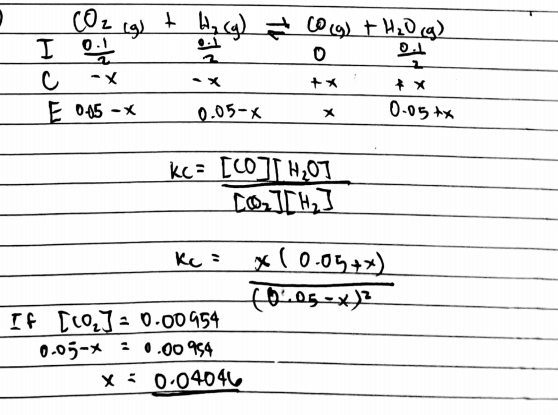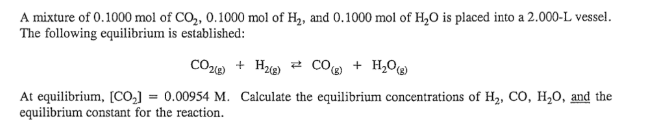# Problem: A mixture of 0.1000 mol of CO2, 0.1000 mol of H2, and 0.1000 mol of H2O is placed into a 2.000-L vessel. The following equilibrium is established: CO2(g) + H2(g) ⇌ CO(g) + H2O(g) At equilibrium, [CO2] = 0.00954 M. Calculate the equilibrium concentrations of H2, CO, H2O, and the equilibrium constant for the reaction.

###### FREE Expert Solution87% (208 ratings)###### Problem Details

A mixture of 0.1000 mol of CO2, 0.1000 mol of H2, and 0.1000 mol of H2O is placed into a 2.000-L vessel. The following equilibrium is established:

CO2(g) + H2(g) ⇌ CO(g) + H2O(g)

At equilibrium, [CO2] = 0.00954 M. Calculate the equilibrium concentrations of H2, CO, H2O, and the equilibrium constant for the reaction.